# Analog signal Conditioning

2 de Apr de 2019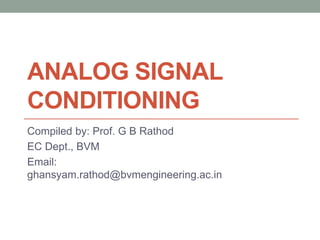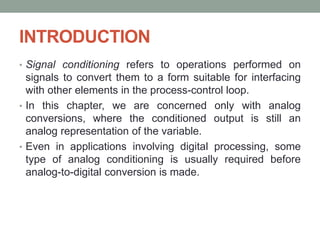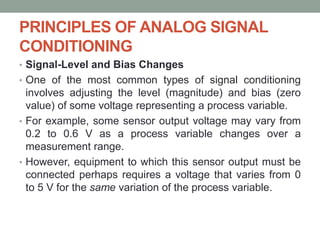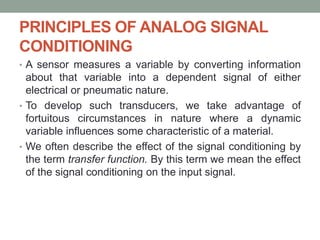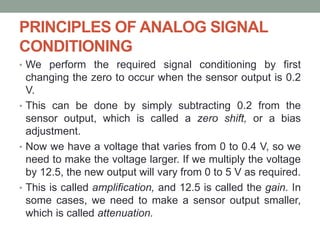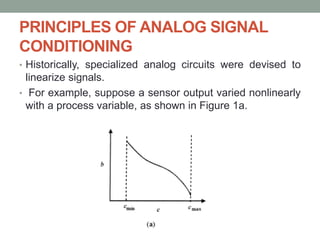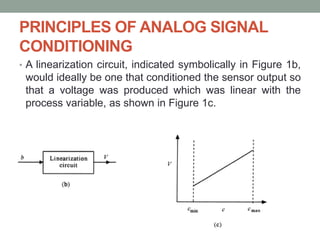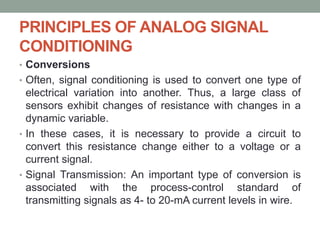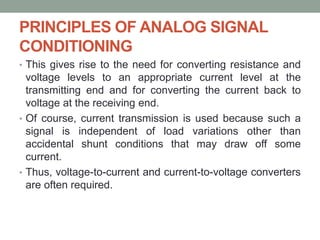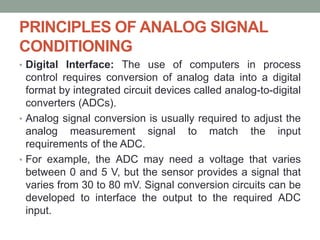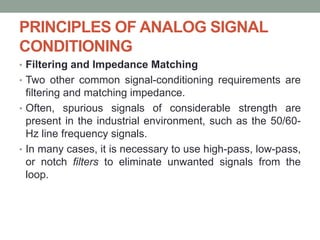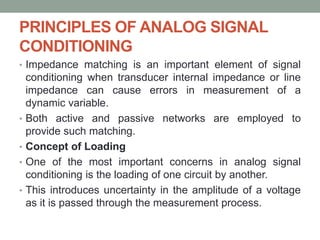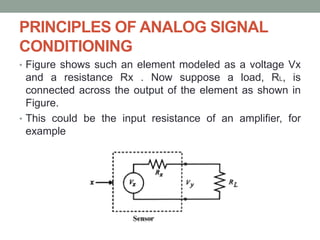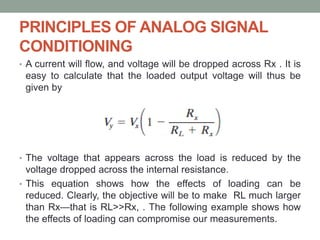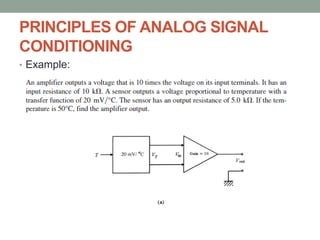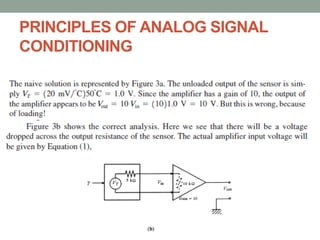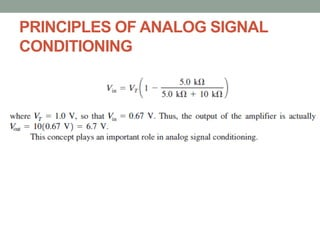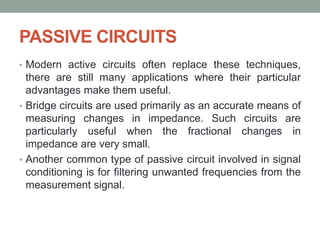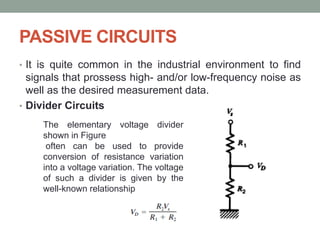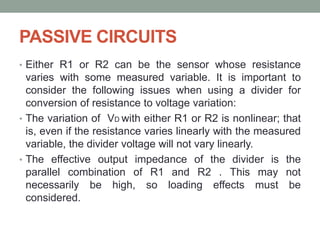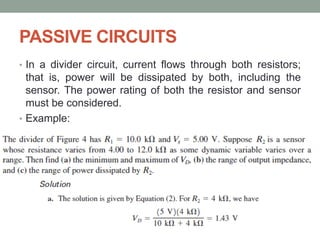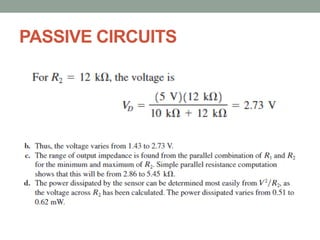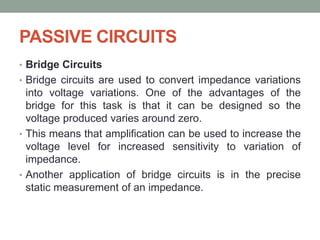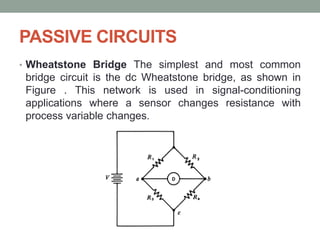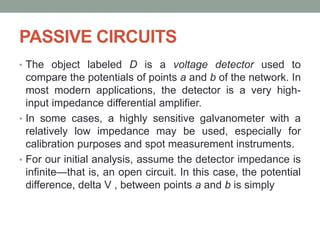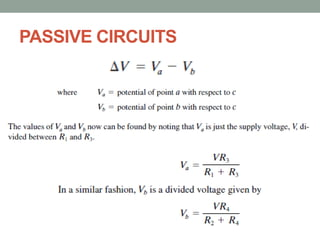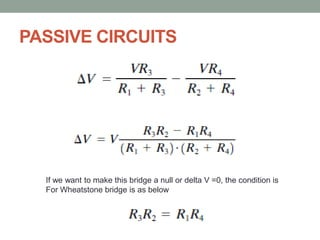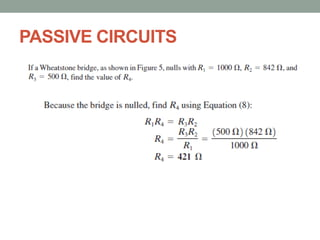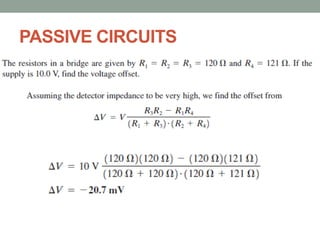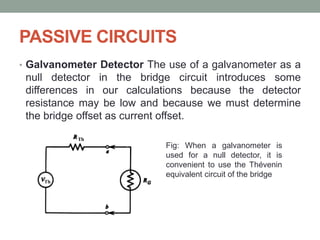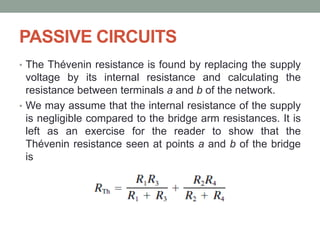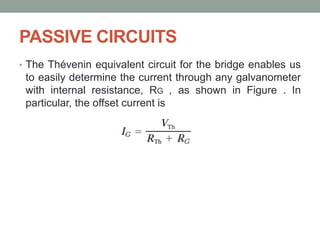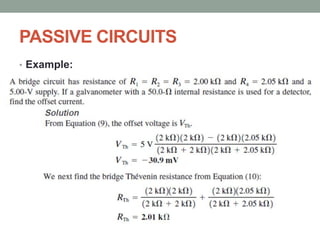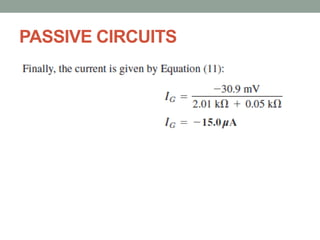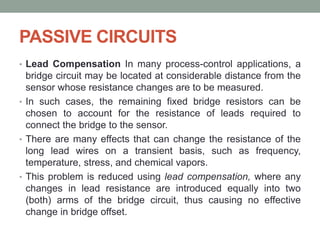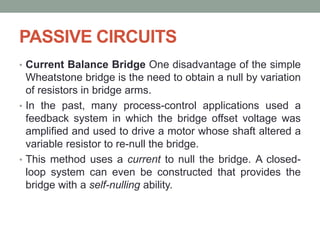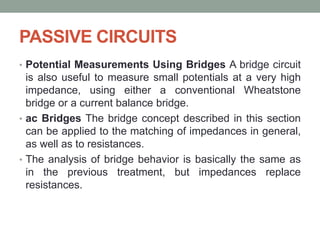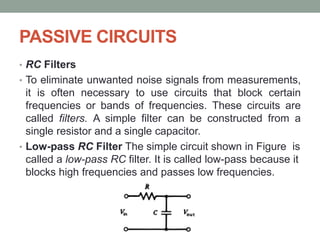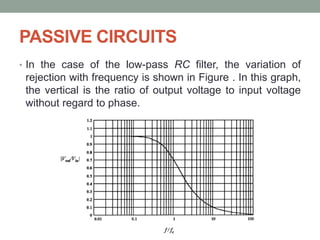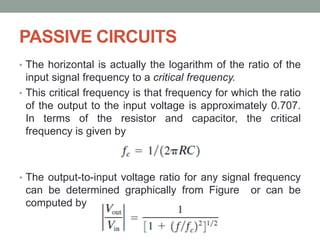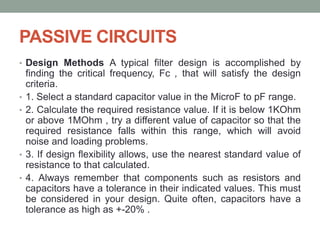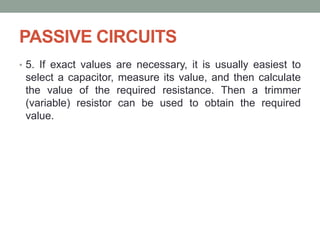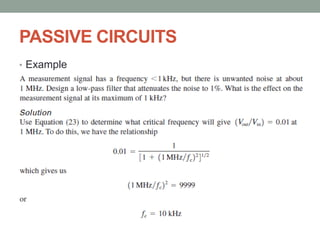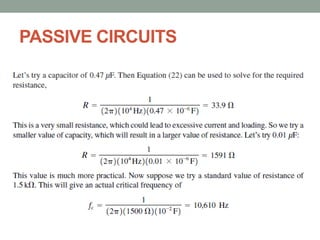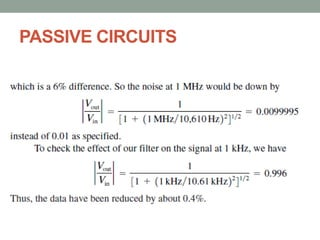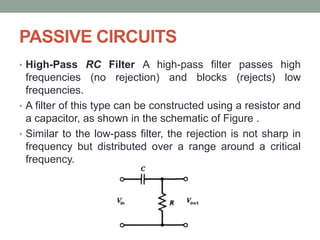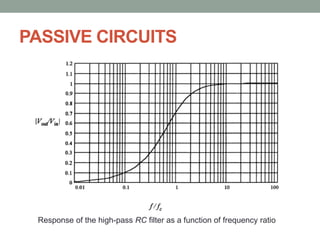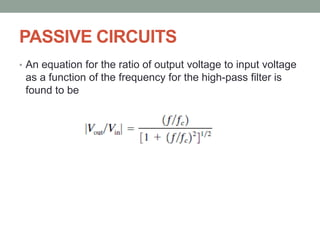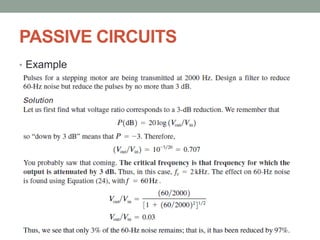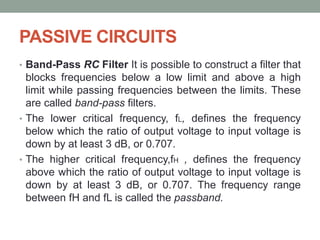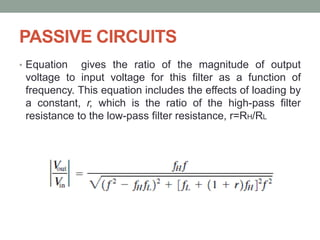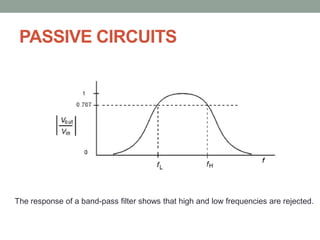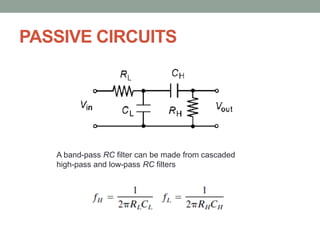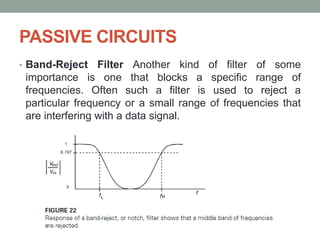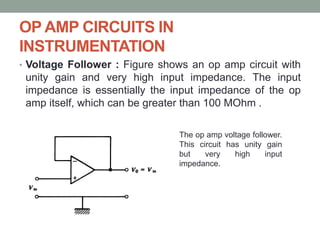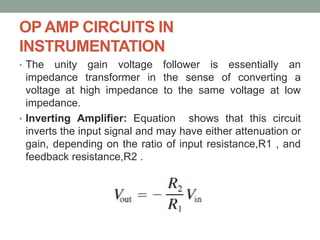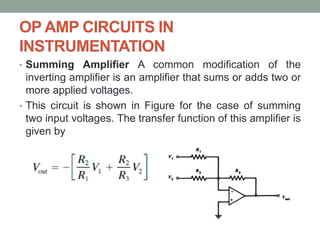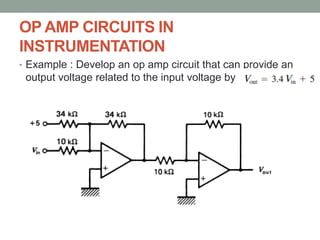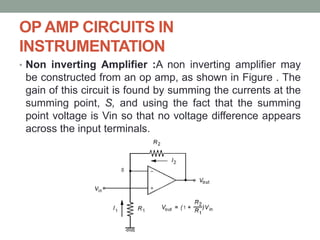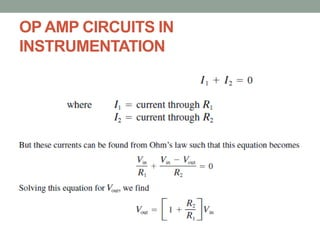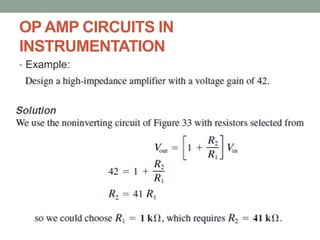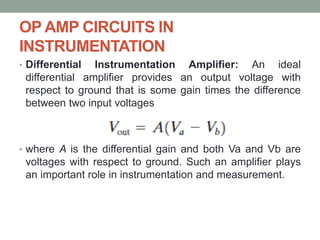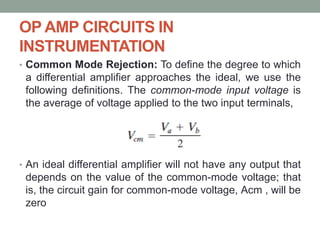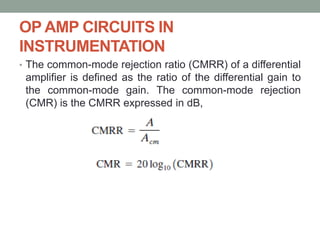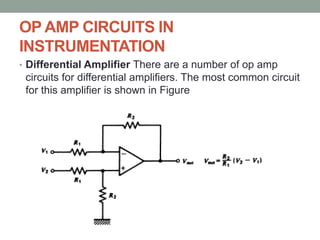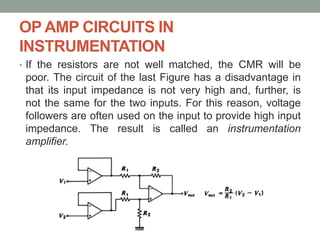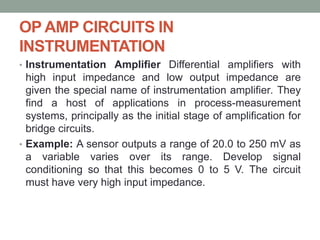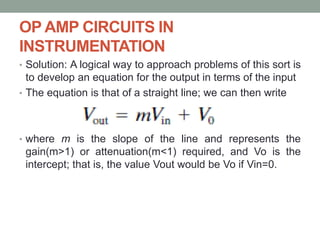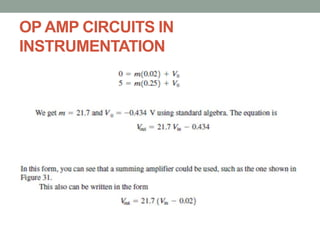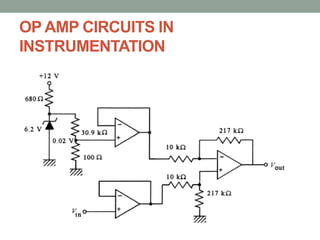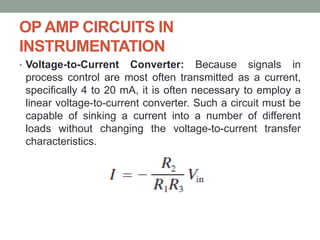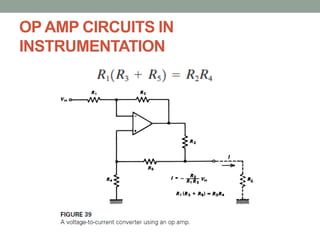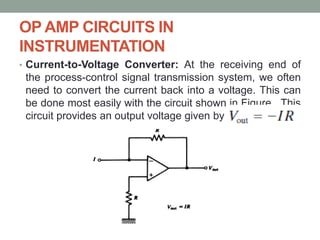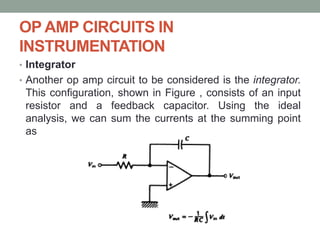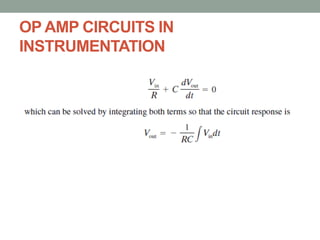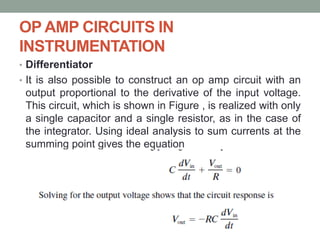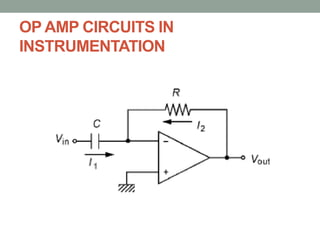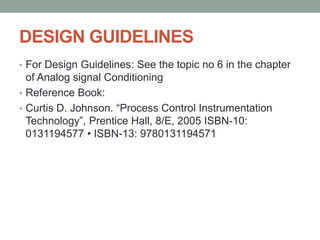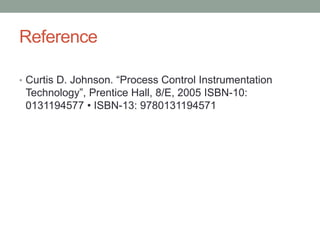1 de 81

### Analog signal Conditioning

• 1. ANALOG SIGNAL CONDITIONING Compiled by: Prof. G B Rathod EC Dept., BVM Email: ghansyam.rathod@bvmengineering.ac.in
• 2. INTRODUCTION • Signal conditioning refers to operations performed on signals to convert them to a form suitable for interfacing with other elements in the process-control loop. • In this chapter, we are concerned only with analog conversions, where the conditioned output is still an analog representation of the variable. • Even in applications involving digital processing, some type of analog conditioning is usually required before analog-to-digital conversion is made.
• 3. PRINCIPLES OF ANALOG SIGNAL CONDITIONING • Signal-Level and Bias Changes • One of the most common types of signal conditioning involves adjusting the level (magnitude) and bias (zero value) of some voltage representing a process variable. • For example, some sensor output voltage may vary from 0.2 to 0.6 V as a process variable changes over a measurement range. • However, equipment to which this sensor output must be connected perhaps requires a voltage that varies from 0 to 5 V for the same variation of the process variable.
• 4. PRINCIPLES OF ANALOG SIGNAL CONDITIONING • A sensor measures a variable by converting information about that variable into a dependent signal of either electrical or pneumatic nature. • To develop such transducers, we take advantage of fortuitous circumstances in nature where a dynamic variable influences some characteristic of a material. • We often describe the effect of the signal conditioning by the term transfer function. By this term we mean the effect of the signal conditioning on the input signal.
• 5. PRINCIPLES OF ANALOG SIGNAL CONDITIONING • We perform the required signal conditioning by first changing the zero to occur when the sensor output is 0.2 V. • This can be done by simply subtracting 0.2 from the sensor output, which is called a zero shift, or a bias adjustment. • Now we have a voltage that varies from 0 to 0.4 V, so we need to make the voltage larger. If we multiply the voltage by 12.5, the new output will vary from 0 to 5 V as required. • This is called amplification, and 12.5 is called the gain. In some cases, we need to make a sensor output smaller, which is called attenuation.
• 6. PRINCIPLES OF ANALOG SIGNAL CONDITIONING • We distinguish between amplification and attenuation by noting whether the gain of the amplifier is greater than or less than unity. • In designing bias and amplifier circuits, we must be concerned with issues such as the frequency response, output impedance, and input impedance. • Linearization • As pointed out at the beginning of this section, the process-control designer has little choice of the characteristics of a sensor output versus a process variable. Often, the dependence that exists between input and output is nonlinear.
• 7. PRINCIPLES OF ANALOG SIGNAL CONDITIONING • Historically, specialized analog circuits were devised to linearize signals. • For example, suppose a sensor output varied nonlinearly with a process variable, as shown in Figure 1a.
• 8. PRINCIPLES OF ANALOG SIGNAL CONDITIONING • A linearization circuit, indicated symbolically in Figure 1b, would ideally be one that conditioned the sensor output so that a voltage was produced which was linear with the process variable, as shown in Figure 1c.
• 9. PRINCIPLES OF ANALOG SIGNAL CONDITIONING • Conversions • Often, signal conditioning is used to convert one type of electrical variation into another. Thus, a large class of sensors exhibit changes of resistance with changes in a dynamic variable. • In these cases, it is necessary to provide a circuit to convert this resistance change either to a voltage or a current signal. • Signal Transmission: An important type of conversion is associated with the process-control standard of transmitting signals as 4- to 20-mA current levels in wire.
• 10. PRINCIPLES OF ANALOG SIGNAL CONDITIONING • This gives rise to the need for converting resistance and voltage levels to an appropriate current level at the transmitting end and for converting the current back to voltage at the receiving end. • Of course, current transmission is used because such a signal is independent of load variations other than accidental shunt conditions that may draw off some current. • Thus, voltage-to-current and current-to-voltage converters are often required.
• 11. PRINCIPLES OF ANALOG SIGNAL CONDITIONING • Digital Interface: The use of computers in process control requires conversion of analog data into a digital format by integrated circuit devices called analog-to-digital converters (ADCs). • Analog signal conversion is usually required to adjust the analog measurement signal to match the input requirements of the ADC. • For example, the ADC may need a voltage that varies between 0 and 5 V, but the sensor provides a signal that varies from 30 to 80 mV. Signal conversion circuits can be developed to interface the output to the required ADC input.
• 12. PRINCIPLES OF ANALOG SIGNAL CONDITIONING • Filtering and Impedance Matching • Two other common signal-conditioning requirements are filtering and matching impedance. • Often, spurious signals of considerable strength are present in the industrial environment, such as the 50/60- Hz line frequency signals. • In many cases, it is necessary to use high-pass, low-pass, or notch filters to eliminate unwanted signals from the loop.
• 13. PRINCIPLES OF ANALOG SIGNAL CONDITIONING • Impedance matching is an important element of signal conditioning when transducer internal impedance or line impedance can cause errors in measurement of a dynamic variable. • Both active and passive networks are employed to provide such matching. • Concept of Loading • One of the most important concerns in analog signal conditioning is the loading of one circuit by another. • This introduces uncertainty in the amplitude of a voltage as it is passed through the measurement process.
• 14. PRINCIPLES OF ANALOG SIGNAL CONDITIONING • Figure shows such an element modeled as a voltage Vx and a resistance Rx . Now suppose a load, RL, is connected across the output of the element as shown in Figure. • This could be the input resistance of an amplifier, for example
• 15. PRINCIPLES OF ANALOG SIGNAL CONDITIONING • A current will flow, and voltage will be dropped across Rx . It is easy to calculate that the loaded output voltage will thus be given by • The voltage that appears across the load is reduced by the voltage dropped across the internal resistance. • This equation shows how the effects of loading can be reduced. Clearly, the objective will be to make RL much larger than Rx—that is RL>>Rx, . The following example shows how the effects of loading can compromise our measurements.
• 16. PRINCIPLES OF ANALOG SIGNAL CONDITIONING • Example:
• 17. PRINCIPLES OF ANALOG SIGNAL CONDITIONING
• 18. PRINCIPLES OF ANALOG SIGNAL CONDITIONING
• 19. PASSIVE CIRCUITS • Modern active circuits often replace these techniques, there are still many applications where their particular advantages make them useful. • Bridge circuits are used primarily as an accurate means of measuring changes in impedance. Such circuits are particularly useful when the fractional changes in impedance are very small. • Another common type of passive circuit involved in signal conditioning is for filtering unwanted frequencies from the measurement signal.
• 20. PASSIVE CIRCUITS • It is quite common in the industrial environment to find signals that prossess high- and/or low-frequency noise as well as the desired measurement data. • Divider Circuits The elementary voltage divider shown in Figure often can be used to provide conversion of resistance variation into a voltage variation. The voltage of such a divider is given by the well-known relationship
• 21. PASSIVE CIRCUITS • Either R1 or R2 can be the sensor whose resistance varies with some measured variable. It is important to consider the following issues when using a divider for conversion of resistance to voltage variation: • The variation of VD with either R1 or R2 is nonlinear; that is, even if the resistance varies linearly with the measured variable, the divider voltage will not vary linearly. • The effective output impedance of the divider is the parallel combination of R1 and R2 . This may not necessarily be high, so loading effects must be considered.
• 22. PASSIVE CIRCUITS • In a divider circuit, current flows through both resistors; that is, power will be dissipated by both, including the sensor. The power rating of both the resistor and sensor must be considered. • Example:
• 24. PASSIVE CIRCUITS • Bridge Circuits • Bridge circuits are used to convert impedance variations into voltage variations. One of the advantages of the bridge for this task is that it can be designed so the voltage produced varies around zero. • This means that amplification can be used to increase the voltage level for increased sensitivity to variation of impedance. • Another application of bridge circuits is in the precise static measurement of an impedance.
• 25. PASSIVE CIRCUITS • Wheatstone Bridge The simplest and most common bridge circuit is the dc Wheatstone bridge, as shown in Figure . This network is used in signal-conditioning applications where a sensor changes resistance with process variable changes.
• 26. PASSIVE CIRCUITS • The object labeled D is a voltage detector used to compare the potentials of points a and b of the network. In most modern applications, the detector is a very high- input impedance differential amplifier. • In some cases, a highly sensitive galvanometer with a relatively low impedance may be used, especially for calibration purposes and spot measurement instruments. • For our initial analysis, assume the detector impedance is infinite—that is, an open circuit. In this case, the potential difference, delta V , between points a and b is simply
• 28. PASSIVE CIRCUITS If we want to make this bridge a null or delta V =0, the condition is For Wheatstone bridge is as below
• 31. PASSIVE CIRCUITS • Galvanometer Detector The use of a galvanometer as a null detector in the bridge circuit introduces some differences in our calculations because the detector resistance may be low and because we must determine the bridge offset as current offset. Fig: When a galvanometer is used for a null detector, it is convenient to use the Thévenin equivalent circuit of the bridge
• 32. PASSIVE CIRCUITS • The Thévenin resistance is found by replacing the supply voltage by its internal resistance and calculating the resistance between terminals a and b of the network. • We may assume that the internal resistance of the supply is negligible compared to the bridge arm resistances. It is left as an exercise for the reader to show that the Thévenin resistance seen at points a and b of the bridge is
• 33. PASSIVE CIRCUITS • The Thévenin equivalent circuit for the bridge enables us to easily determine the current through any galvanometer with internal resistance, RG , as shown in Figure . In particular, the offset current is
• 36. PASSIVE CIRCUITS • Lead Compensation In many process-control applications, a bridge circuit may be located at considerable distance from the sensor whose resistance changes are to be measured. • In such cases, the remaining fixed bridge resistors can be chosen to account for the resistance of leads required to connect the bridge to the sensor. • There are many effects that can change the resistance of the long lead wires on a transient basis, such as frequency, temperature, stress, and chemical vapors. • This problem is reduced using lead compensation, where any changes in lead resistance are introduced equally into two (both) arms of the bridge circuit, thus causing no effective change in bridge offset.
• 37. PASSIVE CIRCUITS • Current Balance Bridge One disadvantage of the simple Wheatstone bridge is the need to obtain a null by variation of resistors in bridge arms. • In the past, many process-control applications used a feedback system in which the bridge offset voltage was amplified and used to drive a motor whose shaft altered a variable resistor to re-null the bridge. • This method uses a current to null the bridge. A closed- loop system can even be constructed that provides the bridge with a self-nulling ability.
• 38. PASSIVE CIRCUITS • Potential Measurements Using Bridges A bridge circuit is also useful to measure small potentials at a very high impedance, using either a conventional Wheatstone bridge or a current balance bridge. • ac Bridges The bridge concept described in this section can be applied to the matching of impedances in general, as well as to resistances. • The analysis of bridge behavior is basically the same as in the previous treatment, but impedances replace resistances.
• 39. PASSIVE CIRCUITS • RC Filters • To eliminate unwanted noise signals from measurements, it is often necessary to use circuits that block certain frequencies or bands of frequencies. These circuits are called filters. A simple filter can be constructed from a single resistor and a single capacitor. • Low-pass RC Filter The simple circuit shown in Figure is called a low-pass RC filter. It is called low-pass because it blocks high frequencies and passes low frequencies.
• 40. PASSIVE CIRCUITS • In the case of the low-pass RC filter, the variation of rejection with frequency is shown in Figure . In this graph, the vertical is the ratio of output voltage to input voltage without regard to phase.
• 41. PASSIVE CIRCUITS • The horizontal is actually the logarithm of the ratio of the input signal frequency to a critical frequency. • This critical frequency is that frequency for which the ratio of the output to the input voltage is approximately 0.707. In terms of the resistor and capacitor, the critical frequency is given by • The output-to-input voltage ratio for any signal frequency can be determined graphically from Figure or can be computed by
• 42. PASSIVE CIRCUITS • Design Methods A typical filter design is accomplished by finding the critical frequency, Fc , that will satisfy the design criteria. • 1. Select a standard capacitor value in the MicroF to pF range. • 2. Calculate the required resistance value. If it is below 1KOhm or above 1MOhm , try a different value of capacitor so that the required resistance falls within this range, which will avoid noise and loading problems. • 3. If design flexibility allows, use the nearest standard value of resistance to that calculated. • 4. Always remember that components such as resistors and capacitors have a tolerance in their indicated values. This must be considered in your design. Quite often, capacitors have a tolerance as high as +-20% .
• 43. PASSIVE CIRCUITS • 5. If exact values are necessary, it is usually easiest to select a capacitor, measure its value, and then calculate the value of the required resistance. Then a trimmer (variable) resistor can be used to obtain the required value.
• 47. PASSIVE CIRCUITS • High-Pass RC Filter A high-pass filter passes high frequencies (no rejection) and blocks (rejects) low frequencies. • A filter of this type can be constructed using a resistor and a capacitor, as shown in the schematic of Figure . • Similar to the low-pass filter, the rejection is not sharp in frequency but distributed over a range around a critical frequency.
• 48. PASSIVE CIRCUITS Response of the high-pass RC filter as a function of frequency ratio
• 49. PASSIVE CIRCUITS • An equation for the ratio of output voltage to input voltage as a function of the frequency for the high-pass filter is found to be
• 51. PASSIVE CIRCUITS • Band-Pass RC Filter It is possible to construct a filter that blocks frequencies below a low limit and above a high limit while passing frequencies between the limits. These are called band-pass filters. • The lower critical frequency, fL, defines the frequency below which the ratio of output voltage to input voltage is down by at least 3 dB, or 0.707. • The higher critical frequency,fH , defines the frequency above which the ratio of output voltage to input voltage is down by at least 3 dB, or 0.707. The frequency range between fH and fL is called the passband.
• 52. PASSIVE CIRCUITS • Equation gives the ratio of the magnitude of output voltage to input voltage for this filter as a function of frequency. This equation includes the effects of loading by a constant, r, which is the ratio of the high-pass filter resistance to the low-pass filter resistance, r=RH/RL
• 53. PASSIVE CIRCUITS The response of a band-pass filter shows that high and low frequencies are rejected.
• 54. PASSIVE CIRCUITS A band-pass RC filter can be made from cascaded high-pass and low-pass RC filters
• 55. PASSIVE CIRCUITS • Band-Reject Filter Another kind of filter of some importance is one that blocks a specific range of frequencies. Often such a filter is used to reject a particular frequency or a small range of frequencies that are interfering with a data signal.
• 56. OP AMP CIRCUITS IN INSTRUMENTATION • Voltage Follower : Figure shows an op amp circuit with unity gain and very high input impedance. The input impedance is essentially the input impedance of the op amp itself, which can be greater than 100 MOhm . The op amp voltage follower. This circuit has unity gain but very high input impedance.
• 57. OP AMP CIRCUITS IN INSTRUMENTATION • The unity gain voltage follower is essentially an impedance transformer in the sense of converting a voltage at high impedance to the same voltage at low impedance. • Inverting Amplifier: Equation shows that this circuit inverts the input signal and may have either attenuation or gain, depending on the ratio of input resistance,R1 , and feedback resistance,R2 .
• 58. OP AMP CIRCUITS IN INSTRUMENTATION • Summing Amplifier A common modification of the inverting amplifier is an amplifier that sums or adds two or more applied voltages. • This circuit is shown in Figure for the case of summing two input voltages. The transfer function of this amplifier is given by
• 59. OP AMP CIRCUITS IN INSTRUMENTATION • Example : Develop an op amp circuit that can provide an output voltage related to the input voltage by
• 60. OP AMP CIRCUITS IN INSTRUMENTATION • Non inverting Amplifier :A non inverting amplifier may be constructed from an op amp, as shown in Figure . The gain of this circuit is found by summing the currents at the summing point, S, and using the fact that the summing point voltage is Vin so that no voltage difference appears across the input terminals.
• 61. OP AMP CIRCUITS IN INSTRUMENTATION
• 62. OP AMP CIRCUITS IN INSTRUMENTATION • Example:
• 63. OP AMP CIRCUITS IN INSTRUMENTATION • Differential Instrumentation Amplifier: An ideal differential amplifier provides an output voltage with respect to ground that is some gain times the difference between two input voltages • where A is the differential gain and both Va and Vb are voltages with respect to ground. Such an amplifier plays an important role in instrumentation and measurement.
• 64. OP AMP CIRCUITS IN INSTRUMENTATION • Common Mode Rejection: To define the degree to which a differential amplifier approaches the ideal, we use the following definitions. The common-mode input voltage is the average of voltage applied to the two input terminals, • An ideal differential amplifier will not have any output that depends on the value of the common-mode voltage; that is, the circuit gain for common-mode voltage, Acm , will be zero
• 65. OP AMP CIRCUITS IN INSTRUMENTATION • The common-mode rejection ratio (CMRR) of a differential amplifier is defined as the ratio of the differential gain to the common-mode gain. The common-mode rejection (CMR) is the CMRR expressed in dB,
• 66. OP AMP CIRCUITS IN INSTRUMENTATION • Differential Amplifier There are a number of op amp circuits for differential amplifiers. The most common circuit for this amplifier is shown in Figure
• 67. OP AMP CIRCUITS IN INSTRUMENTATION • If the resistors are not well matched, the CMR will be poor. The circuit of the last Figure has a disadvantage in that its input impedance is not very high and, further, is not the same for the two inputs. For this reason, voltage followers are often used on the input to provide high input impedance. The result is called an instrumentation amplifier.
• 68. OP AMP CIRCUITS IN INSTRUMENTATION • Instrumentation Amplifier Differential amplifiers with high input impedance and low output impedance are given the special name of instrumentation amplifier. They find a host of applications in process-measurement systems, principally as the initial stage of amplification for bridge circuits. • Example: A sensor outputs a range of 20.0 to 250 mV as a variable varies over its range. Develop signal conditioning so that this becomes 0 to 5 V. The circuit must have very high input impedance.
• 69. OP AMP CIRCUITS IN INSTRUMENTATION • Solution: A logical way to approach problems of this sort is to develop an equation for the output in terms of the input • The equation is that of a straight line; we can then write • where m is the slope of the line and represents the gain(m>1) or attenuation(m<1) required, and Vo is the intercept; that is, the value Vout would be Vo if Vin=0.
• 70. OP AMP CIRCUITS IN INSTRUMENTATION
• 71. OP AMP CIRCUITS IN INSTRUMENTATION
• 72. OP AMP CIRCUITS IN INSTRUMENTATION • Voltage-to-Current Converter: Because signals in process control are most often transmitted as a current, specifically 4 to 20 mA, it is often necessary to employ a linear voltage-to-current converter. Such a circuit must be capable of sinking a current into a number of different loads without changing the voltage-to-current transfer characteristics.
• 73. OP AMP CIRCUITS IN INSTRUMENTATION
• 74. OP AMP CIRCUITS IN INSTRUMENTATION • Current-to-Voltage Converter: At the receiving end of the process-control signal transmission system, we often need to convert the current back into a voltage. This can be done most easily with the circuit shown in Figure . This circuit provides an output voltage given by
• 75. OP AMP CIRCUITS IN INSTRUMENTATION • Integrator • Another op amp circuit to be considered is the integrator. This configuration, shown in Figure , consists of an input resistor and a feedback capacitor. Using the ideal analysis, we can sum the currents at the summing point as
• 76. OP AMP CIRCUITS IN INSTRUMENTATION
• 77. OP AMP CIRCUITS IN INSTRUMENTATION • Differentiator • It is also possible to construct an op amp circuit with an output proportional to the derivative of the input voltage. This circuit, which is shown in Figure , is realized with only a single capacitor and a single resistor, as in the case of the integrator. Using ideal analysis to sum currents at the summing point gives the equation
• 78. OP AMP CIRCUITS IN INSTRUMENTATION
• 79. DESIGN GUIDELINES • For Design Guidelines: See the topic no 6 in the chapter of Analog signal Conditioning • Reference Book: • Curtis D. Johnson. “Process Control Instrumentation Technology”, Prentice Hall, 8/E, 2005 ISBN-10: 0131194577 • ISBN-13: 9780131194571
• 80. Reference • Curtis D. Johnson. “Process Control Instrumentation Technology”, Prentice Hall, 8/E, 2005 ISBN-10: 0131194577 • ISBN-13: 9780131194571